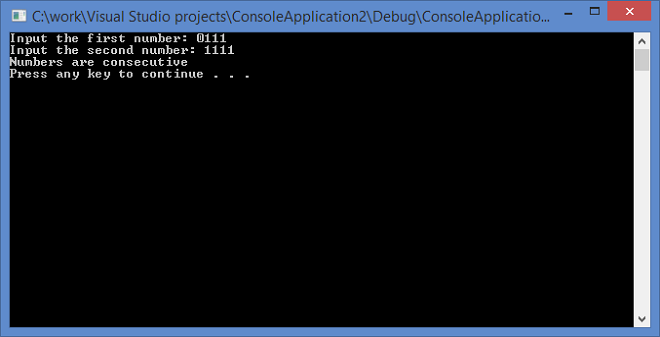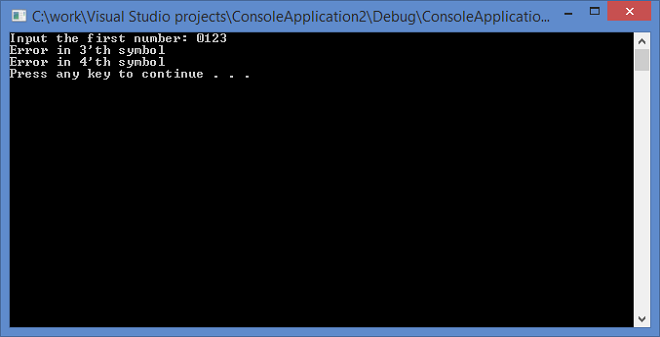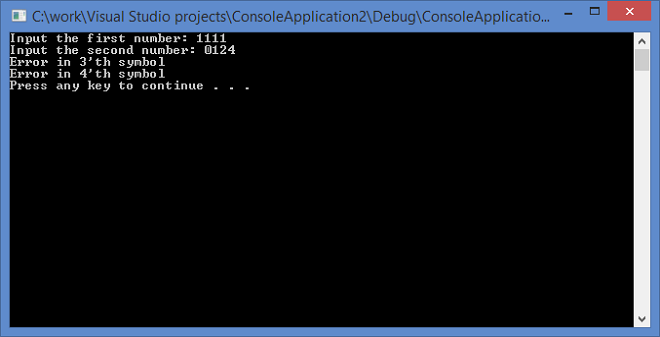# Sample Projects in C#: Consecutive Numbers in Gray Code Sequence

Consecutive Numbers in Gray Code Sequence

• The reflected binary code (RBC), also known as Gray code after Frank Gray, is a binary numeral system where two successive values differ in only one bit (binary digit). The reflected binary code was originally designed to prevent spurious output from electromechanical switches. Today, Gray codes are widely used to facilitate error correction in digital communications such as digital terrestrial television and some cable TV systems. (en.wikipedia.org, 2002)

• So we need to implement a program in C++ that will successively read two numbers which are represented as four-bit numbers. And then it should determine whether these to numbers are consecutive in the Gray code.
• Two binary reflected Gray code neighbors differ by only one bit. But, it doesn’t mean that two Gray codes differing by one bit are neighbors (a => b does not mean that b => a).
• To determine whether two Gray codes are neighbors, we have to check whether previous(a) = b OR next(a) = b. For a given Gray code, you get one neighbor by flipping the rightmost bit and the other neighbor bit by flipping the bit at the left of the rightmost set bit. For the Gray code 1010, the neighbors are 1011 and 1110 (1000 is not one of them).
• Whether you get the previous or the next neighbor by flipping one of these bits actually depends on the parity of the Gray code. However, since we want both neighbors, we don’t have to check for parity.

Screenshots of working program:```#include <iostream>

const int N = 5;//amount of sybmols in the number

bool checkInput(char arr[])
{
bool error = 0;
for (int i = 0; i < 4; i++)
{
if ((arr[i] != '1') && (arr[i] != '0'))
{
std::cout << "Error in " << i + 1 << "'th symbol\n";
error = true;
}
}

return error;
}

void checkGray(char a[], char b[])
{
int sum2(0), sum1(0);

for (int i = 0; i < 4; i++) {
sum1 += a[i];
sum2 += b[i];
}

if (sum1 == sum2 + 1 || sum2 == sum1 + 1)std::cout << "Numbers are consecutive\n";
else std::cout << "Numbers aren't consecutive";
}

int main()
{

char a[N], b[N];//Gray codes

std::cout << "Input the first number: ";

if (checkInput(a) == 1)//check for only 0 and 1 in the number
{
system("pause");
exit(1);
}

std::cout << "Input the second number: ";

if (checkInput(b) == 1)//check for only 0 and 1 in the number
{
system("pause");
exit(1);
}

checkGray(a, b);//check for consecutive numbers

system("pause");

return 0;
}
```

Reference

Gray code. (2002, April 27). Retrieved July 08, 2016, from https://en.wikipedia.org/wiki/Gray_code

Our sample projects in C# were completed just for demonstration of our possibilities, so you shouldn’t use it as your work. You can also enjoy reading one of our C# programming code examples. Better make the order right now and be satisfied with your assignment!

If you need to complete something similar to our sample projects in C# and you don’t know how to cope with this don’t panic. AssignmentShark was established right for students like you. Our experts are able to provide you with qualified assignment help any time you need it. The only thing you need to do is to specify your requirements accurately. During the whole process you’ll be able to keep in touch with your expert via live chat. Also, you have the ability to track order’s progress.